Courses

# Chemistry Test 7 - Electrochemistry, P-Block (Halogen And Noble Gas), Biomolecules, Polymers And Chemistry In Action

## 30 Questions MCQ Test JEE Main Mock Test Series 2020 & Previous Year Papers | Chemistry Test 7 - Electrochemistry, P-Block (Halogen And Noble Gas), Biomolecules, Polymers And Chemistry In Action

Description
This mock test of Chemistry Test 7 - Electrochemistry, P-Block (Halogen And Noble Gas), Biomolecules, Polymers And Chemistry In Action for JEE helps you for every JEE entrance exam. This contains 30 Multiple Choice Questions for JEE Chemistry Test 7 - Electrochemistry, P-Block (Halogen And Noble Gas), Biomolecules, Polymers And Chemistry In Action (mcq) to study with solutions a complete question bank. The solved questions answers in this Chemistry Test 7 - Electrochemistry, P-Block (Halogen And Noble Gas), Biomolecules, Polymers And Chemistry In Action quiz give you a good mix of easy questions and tough questions. JEE students definitely take this Chemistry Test 7 - Electrochemistry, P-Block (Halogen And Noble Gas), Biomolecules, Polymers And Chemistry In Action exercise for a better result in the exam. You can find other Chemistry Test 7 - Electrochemistry, P-Block (Halogen And Noble Gas), Biomolecules, Polymers And Chemistry In Action extra questions, long questions & short questions for JEE on EduRev as well by searching above.
QUESTION: 1

### To which class of compounds do enzymes usually belong ?

Solution: Among the organic macromolecules, enzymes belong in the category of proteins. Proteins are distinct from carbohydrates, nucleic acids and lipids in that a protein is made of amino acids. Amino acids link together into a chain that can fold into a three-dimensional shape.
QUESTION: 2

Solution:
QUESTION: 3

### Night-blindness may develop due to the defciency of

Solution:
QUESTION: 4
The reaction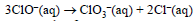is an example of
Solution:
QUESTION: 5
Van der Waals force is the strongest in
Solution:
QUESTION: 6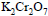reacts with concetrated HCl to produce

Solution:
QUESTION: 7

XeF4 is prepared by heating Xe and F2 at 400oC in the ratio of

Solution:
QUESTION: 8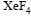reacts violently with water to give
Solution:
QUESTION: 9
Xenon trioxide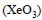has a
Solution:
QUESTION: 10
The chemical name of aspirin is
Solution:
QUESTION: 11
Chlorampehnicol is used as an
Solution:
QUESTION: 12
Phenol is commonly used as
Solution:
QUESTION: 13
Which of the following is not a natural polymer ?
Solution:
QUESTION: 14
Natural rubber is a polymer of
Solution:
QUESTION: 15
Orlon in obtained by the polymerization of
Solution:
QUESTION: 16
Glyptal, a highly cross-linked rigid thermosetting resin,is prepared by heating
Solution:
QUESTION: 17
The polymer obtained by condensation polymerization is
Solution:
QUESTION: 18

Which of the following polymer type is not classified on the basis of its application and properties?

Solution:

Synthetic polymers are classified on the basis of their origin and are known as man-made polymers.

QUESTION: 19
The colour of the precipitate formed when a reducing sugar is heated with Fehling’s solution is
Solution:
QUESTION: 20
Which of the following is an amino acids ?
Solution:
QUESTION: 21
Identify the functional group present in the dipeptide formed from alanine only ?
Solution:
QUESTION: 22
The sugar unit present in the nucleotides of RNA is
Solution:
QUESTION: 23
Nucleic acids are called acids mainly because of the presence of
Solution:
QUESTION: 24
The value of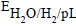at 298K would be
Solution:
QUESTION: 25
The volume of the gas liberated at STP when a current of 5.36 amp is passed through dil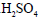for 5 hour will be
Solution:
QUESTION: 26
On electrolysis, which of the following does not give out oxygen ?
Solution:
QUESTION: 27
The standard reduction potentials at 298 K for the half reactions are given against each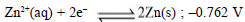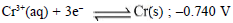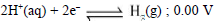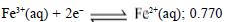Which is the strongest reducing agent ?
Solution:
QUESTION: 28
Standard electrode potentials are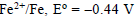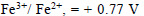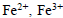and Fe blocks are kept together, then
Solution:
QUESTION: 29
The e.m.f. of the cell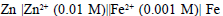at 298 K is 0.02905 volt. The the value of equilibrium constant for the cell reaction is
Solution:
QUESTION: 30
The standard reduction potentials for two reactions are
given below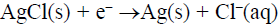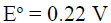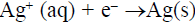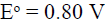The solubility product of AgCl under standard condtions of temperature (298 K) is given by
Solution: